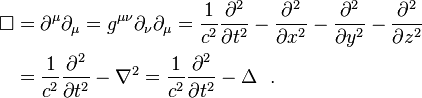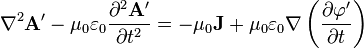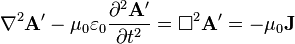# Vector Laplacian: Scalar or Vector?

• I
• olgerm
In summary, the value of Vector_Laplacian is a vector according to the page https://en.wikipedia.org/wiki/Vector_Laplacian, but it is a scalar according to the page https://en.wikipedia.org/wiki/D'Alembertian. The Laplacian operator applied to a vector in Cartesian coordinates is equal to the sum of the second partial derivatives of each component of the vector. This is clarified on the page https://en.wikipedia.org/wiki/Mathematical_descriptions_of_the_electromagnetic_field#Gauge_freedom.

#### olgerm

Gold MemberIs on of these pages wrong or I misunderstand it?

I am asking because I want to know what does Δ2 equal to in thisand thisequation on this https://en.wikipedia.org/wiki/Mathematical_descriptions_of_the_electromagnetic_field#Coulomb_gauge page.

The d'Alembertian is a differential operator. You can apply it to a scalar and get a scalar, or you can apply it to a vector and get a vector.

So since magnetic potential A in this equationis vector ##\nabla^2=(\sum_{i=1}^3(\frac{\partial^2 A}{\partial x_i*\partial x_1});\sum_{i=1}^3(\frac{\partial^2 A}{\partial x_i*\partial x_2});\sum_{i=1}^3(\frac{\partial^2 A}{\partial x_i*\partial x_3}))## not ##\nabla^2=\sum_{i=1}^3(\frac{\partial^2 A}{\partial x_i^2})##?
These are Cartesian coordinates.

It is unclear what you intend to write with these expressions. In particular, it is unclear what you intend for ##A## to be (the vector, the vector components, etc). I strongly suggest that you do not write vectors on component form (x;y;z), but instead use basis vectors. This will make it much clearer what is intended.

The Laplace operator applied to a vector in Cartesian coordinates is such that the ##x## component of ##\nabla^2 \vec A## is equal to ##\nabla^2 A_x##, where ##A_x## is the ##x## component of ##\vec A##.

•olgerm
olgerm said:
A is vector-field. I am trying to find out what does ##\nabla^2 A##, on this Wikipedia page
https://en.wikipedia.org/wiki/Mathematical_descriptions_of_the_electromagnetic_field#Gauge_freedom , equal to.
Is it that ##(\nabla^2 A)_i=\frac{\partial^2 A_i}{\partial x_i^2}## or ##(\nabla^2 A)_j=\sum_{i=1}^3(\frac{\partial^2 A_i}{\partial x_i \cdot \partial x_j})## or ##\nabla^2 A=\sum_{i=1}^3(\frac{\partial^2 A_i}{\partial x_i^2})##?
Where ##A_i## is the ##i## component of ##\vec A##.
Neither, see my previous post.

Your first option is just one term in the sum, your second is grad(div(A)) and not the Laplacian of A, your third is a scalar.

•olgerm
Orodruin said:
Yes. Although this is only valid in Cartesian coordinates.
Ok, thanks.